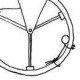# Day 32: Interaction Diagrams and Force DiagramsCollege-Prep Physics: Now that we have gravitational forces, spring forces, and normal forces under our belts, we can analyse more complex situations. Today was a direct instruction lesson* on drawing interaction diagrams and forces diagrams. You might notice some changes from how I drew them from last year.

I’m using agent-object notation on the force diagrams, rather than last year’s force type + agent, in order to combat the misconception that the force diagram represents what the object is doing, rather than what is being done to the object. This also helps with getting the students to focus on the objects that are exerting the forces, because “every force has a source.” To make the force diagrams easier to read and label, we’re not including the force types on the force diagram vectors. Force types are labeled on the interaction diagram only, to help reinforce that a force is a single interaction between objects.

I’m also starting with complex scenarios early, and also asking students to draw more than one force diagram for a given situation. Last year, some students had the misconception that there must always be one force up, down, left, and right. The didn’t realize you could have 2 forces in one direction or no forces at all.

Drawing multiple force diagrams also allows for identifying 3rd Law pairs (the two vectors with circles in #4 above, though we haven’t formally called them 3rd Law pairs).

We also started with numerical values early. Although the scenarios don’t ask a specific question, we determined the values for as many forces as we could based on what was given.

In hopes of avoiding another common misconception, you’ll see that in both scenarios the normal forces aren’t equal to the weights of the objects.

We are only looking at static cases right now. Up next is tension, then friction. After friction, we’ll consider the dynamic cases.

The two scenarios pictured are taken from Preconception in Mechanics, though PiM doesn’t have the students draw interaction diagrams or force diagrams — a fault I found out too late last year. You can get the entire handout here: ForcesSchemaFBDDevelopmentStatic2015

PS: I haven’t been using the HW sheets from PiM at all. Rather, I’ve been using the occasional PiM HW problem as a bell ringer/do now/warm up.

*If you have a more engaging way of introducing interaction diagrams and force diagrams, please share!

##BFPM

NGSS Science and Engineering Practice #2: Developing and Using ModelsHS Physics Teacher constantly questioning my teaching.

### 3 responses to “Day 32: Interaction Diagrams and Force Diagrams”

1.bcphysics says :

Hi Frank,
In BigMarker this morning you mentioned how you have students do a Do Now and then you go over it together. What is the general strategy after a Do Now? Do students work independently on a worksheet (modeling, PiM, your own, whatever), or are you moving on to a new worksheet or concept?

I typically have the class working through a couple of worksheets while I circulate through the room. It gives me enough time to give individual help to everyone. But I wonder if I have the students spend too much time on worksheets. On the other hand, for maybe 1/2 the students, the class is the best time to work on extending the problems because I’m there to help them. It becomes a bit like a tutorial session. My room is terrible for group discussions: its big, and crowded with bolted down benches.

•Frank Noschese says :

Sorry I wasn’t very clear about the Do Now. Sometimes the Do Now is a problem, and when it is, it is sometimes a problem from a worksheet (modeling or my own). I circulate around the room as the kids work. Sometimes I might help kids in the moment until everyone has the correct solution (then we don’t go over it as a class, unless I want to compare solution methods or point out common difficulties groups had). Sometimes I’ll just go over it, or sometimes I’ll call on a group to present.

What comes next depends. The problem’s purpose might have been to get them thinking about a new related concept in that day’s lesson. The problem might have been just to see what they remembered from the day before. In both cases, I’d transition to the day’s lesson. The worksheet itself might be the day’s lesson (like the interaction diagram sheets), so we’ll move on and whiteboard the next problem — hopefully learning from any mistakes made when doing the first problem.

What I’ve found to work well is taking a worksheet and doing each problem as a Do Now over the course of a few days, rather than assign the whole worksheet as HW and go over in class the next day. I think spacing it out over time helps with retention. Also, any mistakes made on the first problem are likely to not reoccur on the 2nd and 3rd one because of the feedback given after each problem. I have effectively done away with modeling’s method of assigning a worksheet for HW and then have the kids whiteboard and present each problem the next day. Sometimes I might have the students start the problem independently and silently for the few minutes of the Do Now, then they can work in groups to finish the problem on whiteboards.

I hope this all make sense. Let me know if you have any questions!